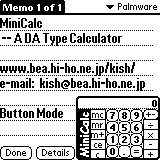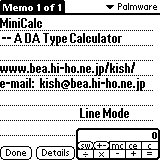## Palmware

\$B;d\$,=q\$\$\$?(JPalm/Pilot\$BMQ\$N%=%U%H%&%'%"\$G\$9!#\$^\$@!"0l\$D\$7\$+\$"\$j\$^\$;\$s\$,!#!#!#\$C\$F\$\$\$D\$^\$G\$?\$C\$F\$b(J1\$B\$D\$G\$9\$M!

Software for Palm/Pilot. This page is mainly written in Japanese, but basic information is also given in English. Is it annoying?

### CalCal\$B%F%-%9%H%U%#!<%k%I\$K%U%)!<%+%9\$,\$J\$\$>l9g\$K%?%\$%H%k\$N2J,\$r%?%C%W\$9\$k\$H(JFatal Error\$B\$H\$J\$k\$H\$\$\$&Js9p\$,B??t4s\$;\$i\$l\$F\$\$\$^\$9!#\$I\$&\$b!"(JOS \$B\$N%P!<%8%g%s\$,(J 3.5 \$B0J9_\$N>l9g\$K\$*\$+\$7\$/\$J\$k\$h\$&\$G\$9!#;DG0\$J\$,\$i%O!<%I%G%#%9%/%H%i%V%k\$G%=!<%9%3!<%I\$,>C\$(\$F\$7\$^\$C\$?\$?\$aBP:v\$,\$7\$?\$\$\$H\$O;W\$\$\$^\$9\$,!"\$\$\$D\$K\$J\$k\$+!#!#!#(J(2001/05/20)

\$B%P%0Js9p\$,\$"\$C\$?\$N\$G=\$@5\$D\$\$\$G\$K5!G=DI2C\$7\$^\$7\$?!#\$&!<\$s!"(JDA\$B\$N\$J\$+\$G\$O\$+\$J\$j\$G\$+\$\$\$G\$9\$M!

CalCal -- \$B\$o\$1\$"\$C\$FL>A0JQ\$(\$^\$7\$?!#(J\$B0JA0\$N\$b\$N(J(MiniCalc)\$B\$O0lEY:o=|\$7\$F\$+\$i%\$%s%9%H!<%k\$7D>\$7\$F\$/\$@\$5\$\$!#\$*\$7\$^\$7\$?!#(J

\$B\$J\$*!"(JDA\$B\$J\$N\$GJL\$K%i%s%A%c!<\$,I,MW\$G\$9!#(JDA\$B\$K\$D\$\$\$F>\\$7\$/\$O(J\$B;3ED\$5\$s\$N%[!<%`%Z!<%8(J\$B\$+;3Lg\$5\$s\$N(JDA\$B\$N>R2p%Z!<%8(J(\$B1Q8l(J)\$B\$r\$4Mw\$/\$@\$5\$\$!#(J